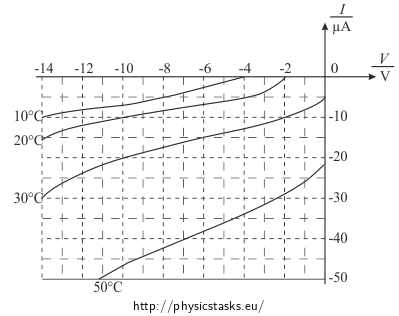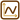## Semiconductor Diode in Reverse Direction

A semiconductor germanium diode is connected into an electric circuit in the reverse direction. A voltage of 10 V is measured across the diode. The figure shows current-voltage characteristics of the diode in the reverse direction for different temperatures of the diode.1. Asses what current flows through the diode at temperatures of 10, 20, 30 and 50 °C.
2. What voltage do we measure across the resistor with a resistance of 5 Ω when the temperature of the diode is 20 °C?

Neglect the current flowing through the voltmeter and the resistance of the ammeter.

• #### Hint for Part a.

We read the values of the current flowing through the diode at a given temperature for a given voltage from the I-V plot of the diode.

• #### Solution of Part a.

The plot indicates the currents through the diode in the reverse direction for the voltage of 10 V for different temperatures of the diode as follows:

 Temperature Current 10 °C 7 µA 20 °C 10 µA 30 °C 20 µA 50 °C 47 µA
• #### Solution of Part b.

The current flowing through the diode at a voltage of 10 V at 20 °C is (see solution of Part a.):

I = 10 µA = 10·10-6 A.

The resistance of the resistor is R = 5 Ω.

The current flowing through the resistor is the same as the current flowing through the diode because they are connected in series.

We calculate the voltage across the resistor from Ohm’s law:

U = RI = 5·10·10-6 V = 5·10-5 V = 50 μV

Currents flowing through the diode at 10 V at different temperatures are:

 Temperature Current 10 °C 7 µA 20 °C 10 µA 30 °C 20 µA 50 °C 47 µA

The voltage across the resistor is 50 μV when the diode is at a temperature of 20 °C.